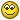# Use of Varibles in Export Scripts

I am attempting to create an export script that uses variables to sum up %length% values as each %track% loop occurs. I am converting the current length to seconds as a \$num(x,n) succesfully, and placing it in a variable. However I still can't find a way to add the next track's %length% value to it. \$add doesn't appear to work on user variables, and there is no real documentation on variables and how they can be used.

If anyone has notes on variable use or could reply with the proper script syntax to do the things below, I would be very grateful.

Generally, Variable1 loaded using \$put(SLEN,(\$num(%_length_seconds%,5))), using Variable2 as the running total, by adding %_length_seconds% or Variable1 with the running sum of the loops.

%add() used with or without \$num does not appear to work.

I could probably use %_total_time_seconds% without modifiacation, but it actually decrements total time by %_length_seconds% on each pass through the loop, which is the opposite of I would expect.

One more confusing area: If I load a variable using \$put(SLEN,(\$num(%_length_seconds%,5))), which results in a \$get value shown as "(nnnnn)", whereas using %_total_time% displays nn:nn:nn.

More confusing is \$num(%_total_time_seconds%,5) which yields 000000

I understand that this is all 'very electric and dangerous,' but if you have a reference or can show an example, it would be much appreciated.For examples see there ...
/t/10145/1
Gesamtlänge eines Ordner in Verzeichnis integrieren
/t/12016/1

DD.20161203.1300.CET

It is not my purpose to find the total playing time of my listed files, but rather to crate a running total.

I.E., Tune #1 length is 90 seconds, Running total is 90 seconds.

``````   Tune #2 length is 208 seconds. Running total is 298 seconds.
Tune #3 length is 104 seconds. Running total is 402 seconds, etc.
``````

I am trying to add the length of the current tune in the loop to the time total of the preceding tunes.

The running total will only show the full running time when the entire loop is completed.

I tried adding \$num(%_length_seconds%,5), to a variable named RTTL, which was created by \$put(RTTL,(\$num(%_length_seconds%,5)) on the first time through the loop.

So far, I have been unable to add a number to an existing (numeric) variable. I can \$add two 'numbers' and \$put them to a variable, but not add a number to value of a numeric variable.

Since the \$get(RTTL) value from the first pass returns the contents in parentheses, I am assuming that it is numeric and that I can use it as a number and add its value in a \$add statement. But it just won't work.

There does not appear to be any explicit functionality for casting a user variable to string or a string to numeric.

If there is any syntactical manipulation that makes it possible to add a numeric value to the value of an existing user variable or put the sum into a second user variable, I would like to know it.

Thank you.Try this:

``````\$loop(%track%)\$puts(RunTotal,\$add(%_length_seconds%,\$get(RunTotal)))%_length_seconds%*\$get(RunTotal)*
\$loopend()``````

Thank you ryerman. I will try as you suggest.

But this raises another question. Why isn't this stuff, (namely the use of '*') documented somewhere? Or am I missing something?

Is this an implicit part of MP3TAG scripting, or is the scripting used by MP3TAG a subset of another scripting language?

If it is, please let me know what language it is based on.so I can refer to it.

Again, thank you for your reply. I hope it resolves the issue."*" has no special meaning here. It did nothing to manipulate data. It is not a wild-card.
I used it merely as a separator for the output, which you will see if you try the export script.
It could have been any other non-special character.

Use the "Support" button at the top of the forum to find lots of documentation.
eg. https://docs.mp3tag.de/scripting

Ryerman,

Thank you again. Your code works fine. It is very similar to what I was doing, using the Scripting References Pages as a guide.

The main difference I can see is that I was using \$num() to convert the output of %_length_seconds% to a numeric value, assuming (incorrectly) that the output would be a string.

You help is greatly appreciated. Thank you!